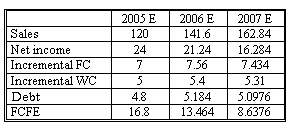### Seeing is believing!

Before you order, simply sign up for a free user account and in seconds you'll be experiencing the best in CFA exam preparation.

##### Subject 3. Forecasting FCFF and FCFE
Several methods exist for forecasting free cash flow.

Method 1: Calculate historical free cash flow and apply constant growth rate.

The easiest method is to assume a constant growth rate that is based on historical trends. This method works best if FCFF grows at a nearly constant rate and has a stable relationship with underlying fundamentals. Obviously, this approach is not very accurate for most companies, as the growth rate of the free cash flow to the firm may vary from year to year.

Method 2: Forecast underlying components of free cash flow: net income, FCInv, dep., WCInv which are tied to sales forecast.

To get a more precise forecast, analysts need to forecast the components of free cash flow by evaluating and capturing the complex relationships among the components of free cash flow. An example of such approach would be forecasting sales and tying them to the components of FCFF. Frequently, a convenient simplifying assumption is made that non-cash charges consist of depreciation expense only. If this assumption holds, FCFF can be calculated as

FCFF = EBIT (1 - Tax rate) - FCInv - WCInv

In the equation above, net investment in new fixed assets is the investment in property, plant and equipment in excess of depreciation.

Often, analysts start with the sales forecast. After that they analyze historical operating profit margin and two ratios:

• Net investment in new fixed assets / sales increase, and
• Investment in working capital / sales increase.

If future values of these ratios are established, the sales forecast is used to arrive at FCFF following the approach outlined above.

To forecast FCFE, practitioners frequently use one additional assumption. Recognize that new net investment in fixed assets and working capital is partly financed by issuance of new debt (net borrowing). If the share of debt in financing of these investments is fairly stable (for example a company has established the target debt to equity ratio), we can express FCFE using the add-back to net income method as

FCFE = NI - (FCInv - Dep) - WCInc + Net borrowing

or,

FCFE = NI - (1 - DR) (FCInv - Dep) - (1 - DR) WCInc

DR: target debt ratio.

This way we can avoid forecasting net borrowing directly. It is enough to forecast sales, net profit margin, and net new investment to arrive at FCFE. If the two assumptions do not hold (e.g., debt ratio is not stable and there are other significant non-cash expenses except depreciation), the forecasts would not be very precise. For that reason, analysts should always conduct research to determine whether there are any planned future investments or changes in capital structure.

Example

Watson wants to forecast FCFE of Alcan, Inc for the year of 2007. The sales amount of Alcan is \$100 million in 2004. After careful research and evaluation, he expects

• sales growth rates to be 20%, 18%, and 15% for 2005, 2006 and 2007, respectively.
• net income margins to be 20%, 15%, and 10% for 2005, 2006 and 2007, respectively.
• the company to finance incremental fixed and working capital investments with 40% debt - the target debt ratio.
• incremental FC (investment in FC - Depreciation) to be 35% of sales increase.
• incremental WC to be 25% of sales increase.

Solution1. Sales = last year's sales x (1 + sales growth rate).
2. Net income = sales x net income margin.
3. Incremental FC = sales growth x 0.35.
4. Incremental WC = sales growth x 0.25.
5. Debt = (Incremental FC + Incremental WC) x 0.4.
6. FCFE = 2 - 3 - 4 + 5.

User Comment
MasterD Net borrowing = DR (FCinv - Dep) + DR WCinv
MasterD Doesn't this form make more sense? Any reason it was split up?

FCFE = NI - (1 - DR)(FCinv - Dep + WCinv)

(assumes Dep is the only NCC)
IBMkeyboard may be they wanted to emphasize on the fact that FCInv and WCInv would be in a such a way that they both maintain the debt2asset ratio...but the equation u wrote would be easier to rememvber.